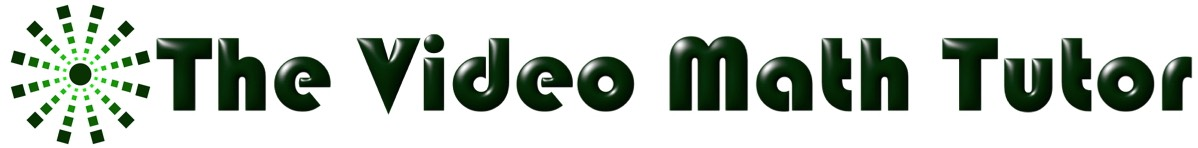• # Welcome!

Open or Close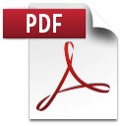Welcome!

This is the place to get all my
FREE Math Notes! I recommend you get these and read BEFORE you watch the video lessons of the same names. This way, I believe you will get more out of each lesson.

Download PDF File" button that appears to first view the notes, then get the notes on that same page (click on: “. . .” in upper right corner of webpage, then select “Download”).
• # Getting Started With The Self-Tutorial Math Notes

Open or Close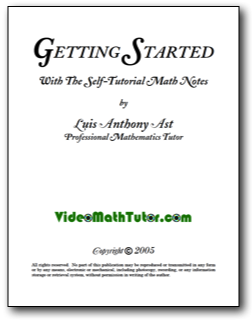Getting Started With The Self-Tutorial Math Notes

Learn how to get started with your math studies and with my notes and videos.

7 pages of printable notes

• # Math Notes Cover Page

Open or Close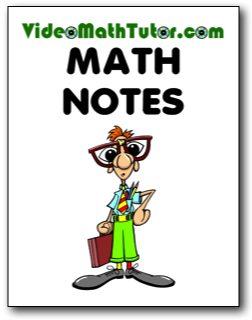Math Notes Cover Page

User this as a cover page for the math notes you get from me, either by watching my videos or my printable math notes.

1 page of printable cover page

• # Alternate Math Notes Cover Page

Open or CloseAlternate Math Notes Cover Page

User this as an alternate cover page for the math notes you get from me, either by watching my videos or my printable math notes.

1 page of printable cover page

• # Basic Math: Lesson 1 - Numbers

Open or CloseBasic Math: Lesson 1 - Numbers

This lesson consists of providing you with a Self-Tutorial on all about the classification and sets of numbers.

12 pages of printable notes

• # Basic Math: Lesson 2 - Equalities & Inequalities

Open or CloseBasic Math: Lesson 2 - Equalities & Inequalities

This lesson consists of providing you with a Self-Tutorial on the basics of equalities and inequalities.

10 pages of printable notes

• # Basic Math: Lesson 3 - Operations On Numbers

Open or Close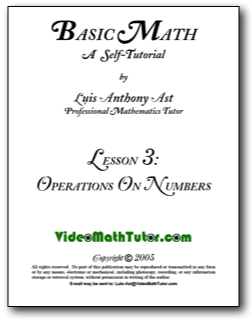Basic Math: Lesson 3 - Operations On Numbers

This lesson consists of providing you with a Self-Tutorial on the typical operations done on numbers.

44 pages of printable notes

• # Basic Math: Lesson 4 - Properties of Numbers

Open or CloseBasic Math: Lesson 4 - Properties of Numbers

This lesson consists of providing you with a Self-Tutorial of the basic properties of numbers.

20 pages of printable notes

• # Basic Math: Lesson 5 - Factors, Multiples & Divisibility

Open or Close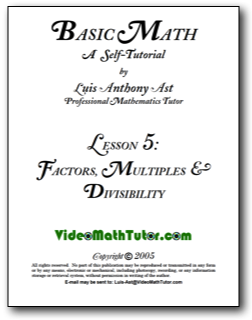Basic Math: Lesson 5 - Factors, Multiples & Divisibility

This lesson consists of giving you the necessary "preliminaries" to do fractions. The tutor explains what are factors, divisors, prime and composite numbers, how to do prime factorization, GCFs, LCMs, multiples, and the rules of divisibility.

19 pages of printable notes

• # Basic Math: Lesson 6 - Fractions

Open or CloseBasic Math: Lesson 6 - Fractions

Lesson 6 consists of providing you with a Self-Tutorial on all about fractions.

83 pages of printable notes

• # Basic Math: Lesson 7 - Units Of Measurement

Open or Close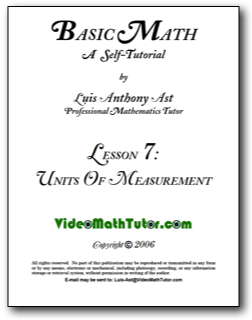Basic Math: Lesson 7 - Units Of Measurement

This lesson consists of providing you with a Self-Tutorial of the basic units used in measurement.

21 pages of printable notes

• # Algebra: Formulas From Geometry

Open or CloseAlgebra: Formulas From Geometry

This lesson consists of providing you with a basic review of the formulas from geometry you will most likely encounter in algebra (and other math classes like trigonometry and calculus). This is NOT a detailed, formal lesson.

10 pages of printable notes